• CFD, Fluid Flow, FEA, Heat/Mass Transfer

Post-processing of CFD Results

### Extracting Engineering Information from CFD Results

Post-processing activity includes generation of detailed report with the help of quantitative data, qualitative data, contour plots, vector plots, streamlines, area-average values, mass-average values, pressure coefficient, lift coefficient, centre of pressure.

One of the commonly used term in post-processing and visualization technique is 'rendering'. This refers to the process of converting underlying mathematical representation of solid geometry into visual forms.

The screen is represented by a 2D array of locations called pixels. One of 2N intensities or colors are associated with each pixel, where N is the number of bits per pixel. Greyscale typically has one byte per pixel, for 28 = 256 intensities. Color often requires 1 byte per channel, with 3 color channels per pixel: red, green, and blue. An "image map" or 'bitmap' or "frame buffer" is a array or variable to store color data. Z-buffer is the element of the computer hardware/software that is expected to manage the depth of the image (in the z-direction - into the plane of the screen).

The important of visual data is summarized in image below. Look at the comparative statistics of information retained by people: 80% in case of visual information, 20% of reading and just 10% of information reaching through ears.Excerpts from ParaView tutorial manual: "the process of visualization is taking raw data and converting it to a form that is viewable and understandable to humans. This allows us to get a better cognitive understanding of our data. Scientific visualization is specifically concerned with the type of data that has a well defined representation in 2D or 3D space. Data that comes from simulation meshes and scanner data is well suited for this type of analysis. There are 3 basic steps to visualizing your data: reading, filtering and rendering. First, your data must be read into ParaView. Next, you may apply any number of filters that process the data to generate, extract or derive features from the data. Finally, a viewable image is rendered from the data."

Checklist for Simulation Result

 No. Checkpoint Record 01 Has the overall mass imbalance of ≤ 0.01% achieved? 02 Do the velocity and pressure profiles at inlets and outlets been checked for uniformity? 03 Has the pressure drop reported for porous domains been checked with expected value as per P-Q curve? 04 Has the contour plot been set as banded instead of continously coloured? 05 Has the precision of labels (number of decimal places) set as per the range of data on legend? 06 Has the format of number labels on legend set as per magnitude of values: float, decimal or exponential? 07 Has the material properties at inlets and outlets checked to be closed to expected and/or specified values?
These are some basic sanity checks which one should make before starting to prepare detailed report. One should always think how easy the plots are to read, interpret and draw conclusions.

#### Vector Plot

A vector plot is qualitative representation of spatial magnitude. The only limitation is that it can be drawn plane or a 3D twisted surface. For any vector or contour plot, one of the important consideration is to selection the number of colour bands (also called the legend).
• This should be small enough to have a distinct interval and high enough to keep it legible and easy to read and distinguish.
• A value between 8 and 16 normally is a good choice.
• Note the example below has 20 bands (with 21 values) and how cluttered it looks. There are 2 colours very close in intensity and cannot be easily distinguished looking at the plots.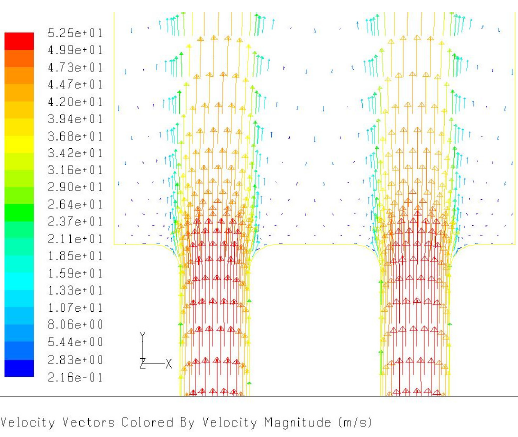#### Streamlines

Streamlines are very good representation of velocity field, at least to beginners in CFD. It is closely related to velocity vector and any inconsistency may arise only because of post-processing interpolation on coarse mesh. As theoretically explained, tangent to streamlines gives direction of velocity field at that point.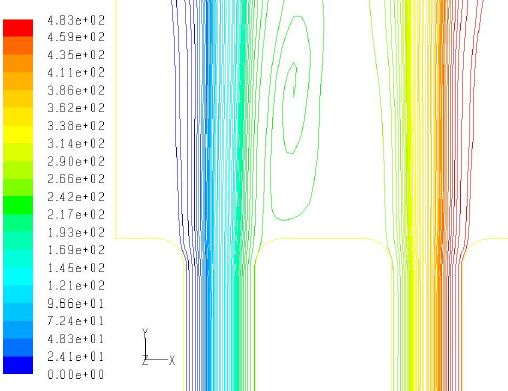#### Contour Plot

Contour plots are "coloured-band" plots of any variable where range of value is represented by a single colour band. This is good presentation of information in both the qualitative and quantitative format.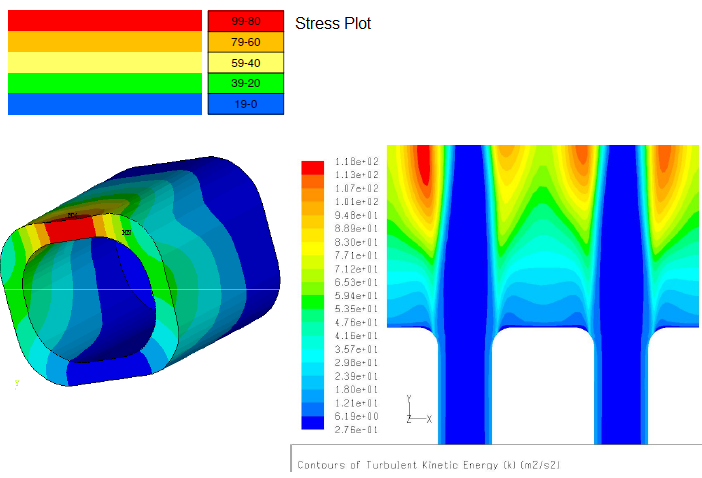#### Iso-surfaces

Iso-surfaces are surface or planes with constant value of a particular variable. CFX-post has feature to create interactively, same feature is available in FLUENT through Iso-surface option. Hence, to create a plane parallel to X-Y plane, Z value will remain constant. Iso-surfaces are also useful to visualize the effect of one variable on any other variable over the entire domain.

#### Mass-weighted or Area-weighted?

The features explained above are more qualitative in nature and may not be used directly in design calculations which usually require a discrete value. This can be obtained by "area-weighted average" or "mass-weighted average" feature available in the post-processing tools. But, the choice of area-weighting or mass-weighting should be based on the gradients of the chosen field variable. For example, to estimate average temperature at a given section for internal flow, mass-weighted option is the correct method as explained below.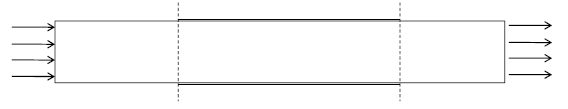In the pipe flow example above, for calculation of temperature at the planes shown by dashed lines, area-weighted option may not give the correct result as it is a function of mesh size near wall. In the example below, area-weighted average velocity at inlet and the two outlets will not be in the ratio of flow areas even though flow is assumed incompressible. This is because of the error in integration or summation due to sharp gradient of velocity in the boundary layer and mesh may not be fine enough to capture it. Also note that the narrower sections have 4 boundary layers as compared to 2 boundary laters in inlet section.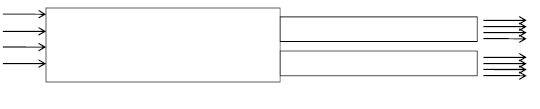Area-weighted average pressure in a fully-developed turbulent flow exit: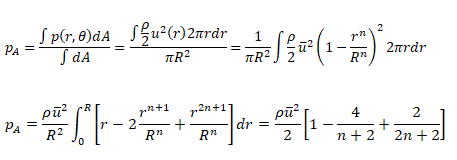Mass-weighted average pressure in a fully-developed turbulent flow exit: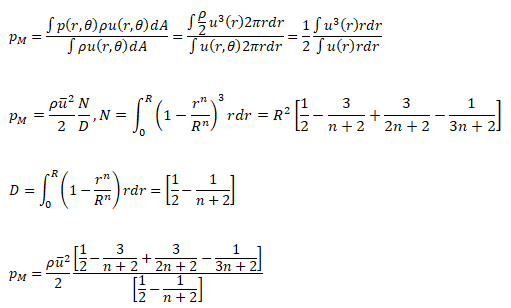Example calculations: let's assume a 5x5 grid with total area of 70 [cm2]. The assumed distribution of velocity, temperature and density for each cell is also described.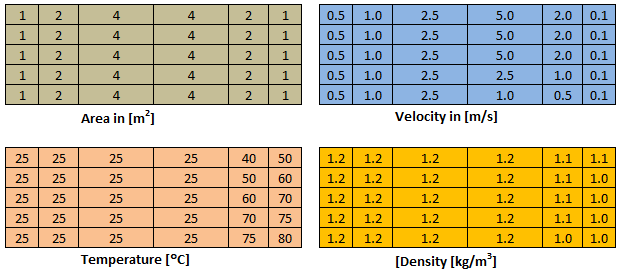For this sample grid, the average velocity is 1.550 [m/s], the area-weighted average velocity = 2.171 [m/s], mass-weighted average velocity = 3.251 [m/s], average temperature = 37.7 [°C], area-averaged temperature = 32.9 [°c] and mass-weighted average temperature = 27.8 [°C]

#### Separation and Re-attachment

There are few post-processing operations which require not only a good insight into the flow physics but experience as well. For example, the estimation of separation length (the reattachment point) needs careful evaluation. There are many methods, one recommended method can be generation of y+ plot. By virtue of re-attachment, the velocity necessarily has to go close to zero and hence y+ or shear stress will follow the same variation. The following image represents y+ plot for flow over back-facing step.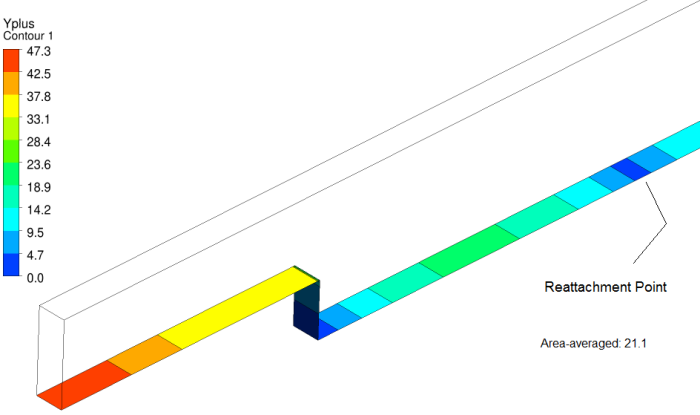Special variables such as Line Integral Convolution Visualization (reference ANSA Training and Brochure Documents):#### General Recommendations for Report Preparation

• One picture or sketch (preferably an isometric or sectional view) representing the extent, origin and axes of computation domain, boundaries and moving walls (if any).
• Sectional view of mesh in area of interest highlighting the boundary layer, growth and orthogonality.
• Mesh quality matrix, worst values of mesh Equi-angle skewness and aspect ratios.
• The description of material properties and its thermodynamic behaviour.
• Tabulated summary of boundary conditions and turbulence parameters.
• Tabulated summary of solver setting: discretization scheme, wall function, relaxation factors
• Contour plots
1. Limit the number of colour bands to 10
2. Set the decimal notation to FLOAT, INTEGER or SCIENTIFIC (exponential) based on mangnitude. E.g. for values ≤ 1000, it is better to use decimal notation istead of exponential format
3. Chose the unit easy to read and interpret: e.g. [K] for temperature is difficult to quicky visualize in mind. For most of us, 37 [°C] which is out body temperature or 25 [°C] which is standard ambient temperature serves as reference
4. Similarly, a value of 0.075 [m] takes more time to interpret than 75 [mm]. It is quicker to deal with integers than fractions

##### Paragraph, Fonts...
1. Chose font size ≥ 11 px, font-type should be easy to read. Calibri, Arial are few good fonts.
2. Use line spacing of 1.50 or higher. For bullet points, it can be reduced to 1.25
3. If paragraphs are not indented, quadruple-space the paragraphs i.e. add extra space before the pargraphs
4. Keep margin of 20 ~ 25 [mm] on each side
5. Add page number centred under footer
6. Maintain uniformity of font sizes such as for Paragraphs, Headers, Captions...
7. Use unique symbols for variables
8. Do not use mix of small letters and capital letters for same variables such as u or U, p or P
9. Use over-dot for mass flow rates such as
10. List standard (Roman) alphabets and Greek alphabets separately
11. Arrange the variable names in alphabetical order
12. Keep a separate list for subscripts and superscripts
13. Add page number in references, typically any documents (books, journals, theses, research papers...) having number of pages ≥ 5
14. Do not use superscript of 'o' or '0' as degree symbol. All of the MS-Office programs Excel, Word and PowerPoint provide degree symbol (°).
15. Do not use underline for words containing g, j, p, q, y
16. Write all units within square brackets [...]
17. No space should be left in front of (before) a punctuation mark
18. It is better to write inline reference with page number (e.g. Sukhatme, 1998, p. 21)
19. For all title of in-text citations, the first letter of every word except articles (a, an, the), prepositions (such as in, on, under...), and conjunctions (such as and, because, but, however ...) should be capitalized, unless they occur at the beginning of the title or subtitle
20. In-text citations must provide the name of the author or authors and the year the source was published
21. The references page should be double-spaced and lists entries in alphabetical order by the author’s last name

Spelling Errors:

1. Note that there is no space before any of the puncutations: . (full stop) , (comma) : (colon) ; (semi-colon) closing ' (apostophe) and closing " (double quote)
2. There are some words which spell check cannot identify as error. There are words generated due to nearby key on the kewords: e.g. [any nay], [out our], [neat near], [near hear], [below bellow], [field filed], [from form], [for fro], [its it's]
3. Check all the occurrences of 'it' and ensure 'it' and 'is' are used appropriately. Note that its and it's are not same
4. Do not use &
5. Full stop is used only at the end of last entry of a bulletted list.

##### Post-processing
In ANSYS FLUENT pre-post, walls and section planes are diplayed along with partition boundaries. To remove the partition boundaries - try (cxg-stitch-shells). This SCHEME command needs to be used after every new operation. Alternatiely, you can try TUI: "define beta yes" followed by "display set duplicate yes".
• Use same lower and upper limits of legends for contour as well as vector plots
• Use decimal notation if variables are > 0.01. Even though scientific notations can be used, it is easier for human mind to read numbers as compared to exponential notations.
• Use number of significant digits judiciously. For example, for most of the industrial applications, it is not important to specify velocity to the 1/10 of mm/s. The number of significant digit is also dependent on the units chosen. For example, 3 decimal places for [Pa] such as 1045.368 [Pa] is irrelevant where as it is a need if unit chosen is [bar] or [kPa] such as 1.034 [kPa]. Followings are more information about "number of significant figures or digits".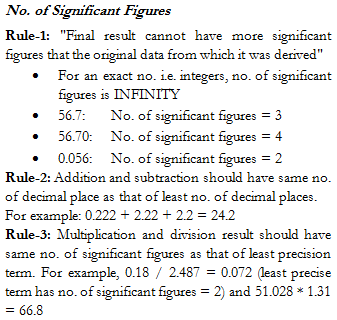##### Recommendations for Rotating Reference frame
• Clearly specify the rotating and stationary domain, direction of rotation, location of the interfaces.
• Show the overlapping view of meshes at the interfaces, if not 1:1.
• Mention the location of the place used to estimate pressure heads developed by the machine. It is further recommended to use 3 or more close locations on the upstream as well as downstream sides to estimate the grand average values of the pressure.
• The physics governing performance of turbo-machines uses many non-dimensional coefficients. Include the plots of important performance parameters such as pressure coefficients on the blades
• On all the plots dealing with flow passage and blades, explicitly mention the suction and pressure sides.
Cell by cell data: A histogram can be generated in ANSYS FLUENT to check cell data. For example, CFL number is important in transient simulations. CFL number can be checked by post-processing operations: Results → Plots → Histogram → Set Up... → Select Velocity... under Histogram of → Select Cell Courant Number from the Velocity... category → Set the value for Divisions to desired value say 50, 100 or 200 → Click Plot.

#### Flux Values

Flux values are important to check the conservation of mass, momentum and energy. Note that in case there are reverse flows at the outlet, the area-weighted average values of temperature and pressure may signficantly deviate from expected value. In other words, the gain in internal energy of fluid as calculated from [mass flow rate] x [specific heat capacity] x [TEX - TIN] may not be equal to the heat gained by the air through the walls and the heat soures. However, this is more of a data interpolation error on finite cells at the outlet and has less implication on the global energy balance. In case of flows with heat transfer, it is important to set the temperature of fluid entering into the computational domain at the outlets (the reverse flows) close to the expected values to reduce the deviation with respect to thermodynamics energy balance described above.

The discrepancies increases with reduction in mass flow rates such as buoyancy-driven flows. Hence, it is important to move the outlet plane to a location where such reverse flows are not expected.

• The mass flow rate through a boundary is computed by summing the [dot product of the density × the velocity vector] and the area projections over the faces of the zone.
• The total moment vector about a specified center of action is computed by summing the [cross products of the pressure and viscous force vectors] for each face with the moment vector.

#### Data Exchange

There is no direct option in any of the commercial software to read results from other commercial software in their native formats. However, most of the software provide options to export data into some common post-processor such as FieldView or Tecplot. The option to export and import data from CGNS format also exist.
##### Export option in FLUENT##### Import option in FLUENT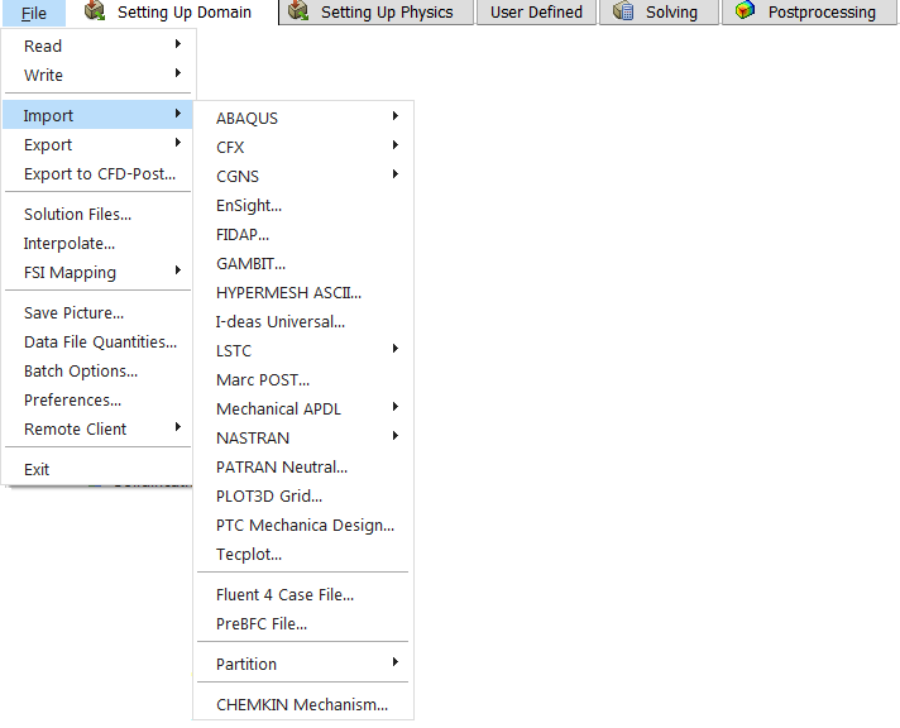##### Import option in STAR-CCM+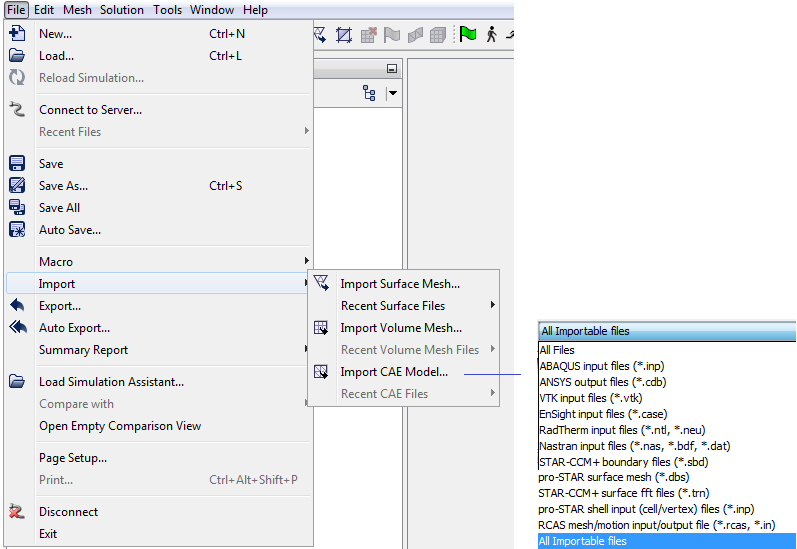#### Centre of Pressure

Centre of pressure - CofP (which depends on the location of each cell and pressure force acting on it) is not same as coefficient of pressure - Cp (which depends on the total pressure force and a arbitrarily chosen reference area. The center of pressure is the point on a body where the total sum of a pressure field acts, causing a force and no moment about that point.

CofP = ∫(x * P.dA)/∫(P.dA) or discretely as ∑(xi * π *Ai)/∑(π * Ai), Cp = ∫(P dA) / AREF

• Let {F} = (Fx, Fy, Fz) and {M} = (Mx, My, Mz)
• Mx = 0*x + Fz*y - Fy*z
• My = -Fz*x + 0 *y + Fx*z
• Mz = Fy*x - Fx*y + 0*z
• As diagonal of the [F] matrix in {M} = [F] {x} is zero, they are singular (i.e. one or more equations are not independent). inv(F) does not exist and det[F] = 0.
• Unit vector in force direction {f} = {F}/|F| = (Fx, Fy, Fz)/|F| where |F| = sqrt(Fx*Fx + Fy*Fy + Fz*Fz)
• Moment parallel to F (pure couple) can be calculated by taking component of {M} along {f}. Thus: {MF} = [{M}.{F}] {f} = (Fx, Fy, Fz) * (Mx*Fx + My*Fy + Mz*Fz) / |F| / |F|
• We need to find a location about which Mz = 0 then using the equations Mz = Fy*x - Fx*y + 0*z we get 0 = Fx*x - Fy*y. Thus, y = (Fx*x)/Fy
• Mz = -Fx *y + Fy * x and y = (Fx*x)/Fy. Thus: Mz = -Fx*(Fx*x)/Fy) + Fy*x = (-Fx2/Fy)*x + Fy*x
• Hence, x = Mz/(-Fx2/Fy + Fy)
• Note: The equations used to calculate the CofP location cannot be used to calculate the moment at the CofP. The moments in those equations are the moments about the origin.

#### Rules of Thumb: Interpolation of Results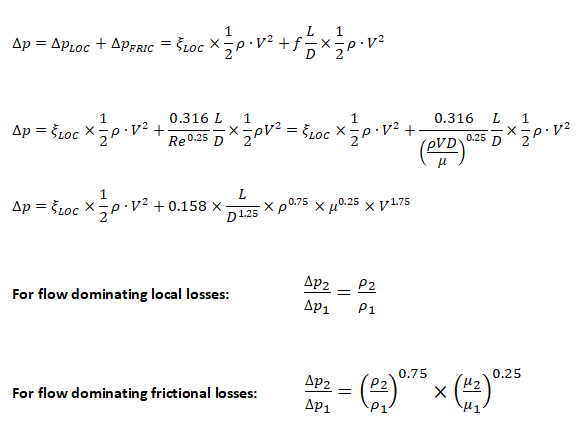The content on CFDyna.com is being constantly refined and improvised with on-the-job experience, testing, and training. Examples might be simplified to improve insight into the physics and basic understanding. Linked pages, articles, references, and examples are constantly reviewed to reduce errors, but we cannot warrant full correctness of all content.